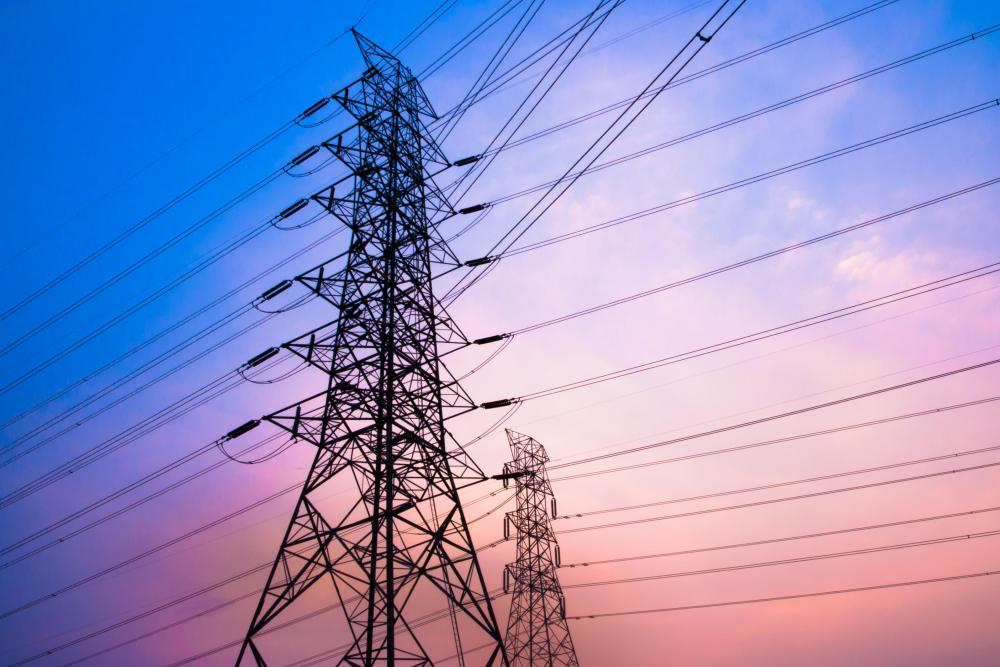# What Limits Electricity Transmission?

Carol Francois

There are several key factors that limit electricity transmission, such as the distance between the generator and the end user, the power of the original transmission, the material used to carry the electricity and the location of transmitters and capacitors. Any of these factors can limit the strength of the electrical power to the end user. All these issues must be carefully monitored at all times to ensure safe transmission of electricity.

As energy is transmitted along a circuit, a certain percentage of the power is lost. This is due to the energy required to move the electricity from the power generation source to the user. The rate of loss is defined in Joule's Law. This law states that the amount of energy lost is in proportion to the squared value of the current voltage.A certain amount of power is lost as energy is transmitted along a circuit.

In order to guarantee the delivery of a specific level of electricity to the end user, electricity is transmitted at a very high voltage. If the voltage is more than 2,000 kilovolts, corona discharge loss must be taken into consideration. The corona discharge loss is the amount of energy lost through the creation of an electric field surrounding the power line as it carries the electricity. This discharge occurs naturally and is the cause of the buzzing sound emitted by high voltage power lines. On average, there is a 7.2% energy loss rate that can be attributed to the movement of electricity and this limits electricity transmission over long distances.

Electricity is transmitted using a set of high voltage cables to carry the electrical current from the power generation station to a series of transformers. These cables are very thick and are designed to withstand the high amount of heat generated by the electricity as it moves through the cables. The heat threshold of the cables is a factor that limits electricity transmission rates. As the volume of electricity being transported along the cables increases, so does the temperature.

Power utilities typically add capacitor banks, phase shifting transformers, and phase conductors in strategic locations to control the power flow, minimize power loss and manage the known issues that limit electricity transmission. The length of uninterrupted power cables has been considerably shortened in an effort to manage the level of energy loss. This change has the added benefit of encouraging the development of a distributed power network. This network reduces the risk of extended power outages over a large area if a particular cable becomes damaged. The outage would be restricted to a smaller area that can be serviced by an alternate power distribution line.

Once the power is received on a household circuit, the electricity can be passed along extension cords to increase the length of the transmission. As energy is transmitted along the cord, a certain percentage of the power is lost. The loss is due to the energy required to move along the distance from the power generation source to the user and it limits electricity transmission.

If the electrical current voltage in a circuit is 110 volts, then the electrical current lost is a factor of 10. To understand this concept, try the following experiment. Attach a standard 100 foot (30.48 meters) power cable and plug it into a lamp with a 100 watt bulb. If you attach nine more 100 foot (30.4 meters) extension cables between the lamp and the power outlet, the total distance that the electricity would have to travel is 1,000 feet (304.8 meters). Due to the amount of electrical current lost while traveling this distance, there would not be enough power available to light the 100 watt bulb.

## You might also Likeanon151072

"If the electrical current voltage is 110 volts..."

Voltage and current are 2 different measurements. Current is the amount of charge that moves past a given point over an amount of time and is measured in Amperes (Coulombs per second). Voltage (a.k.a. potential difference) is the energy per unit charge (Joules per Coulomb, a.k.a. Volt).

If you correct these areas in the last paragraph it would be more clear. Also, don't be afraid to show the math behind this. This is simple algebra that most would be able to comprehend and would find enlightening and useful, both in understanding the paragraph and in uses around the house.

anon42711

So with the last paragraph you are saying that the bulb would not light, not that it would cost more to light the bulb? I found this site by searching the question: does it cost more to operate an appliance by using extension cords.